38 Invariants and Euler-Lagrange-Equations

At the beginning of this chapter, I would like to put a quote: "By generalizing Euler's method of variational calculus, discovered by Lagrange [1736-1813], he showed how to write in a single line the basic equation for all the problems of analytical mechanics." (Quote by Carl Gustav Jacob Jacobi, 1804-1851). The calculus of variations implies in principle that real physical systems move on stable orbits: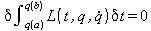(37.1)

The variation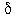means a variation of the orbital parameters on the track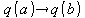. The execution of the variation leads to the Euler-Lagrange equations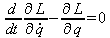(37.2)

which are a condition for the solution. The positions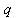and velocitiesare the socalled generalized coordinates, which need not necessarily be the usual location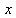and speed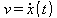. This fundamental principle was later further enhanced, particularly by Emmy Noether80 (1882-1935).

In practical cases, one needs to apply the algorithm to a global conserved quantity of the system under consideration in order to derive the equations determining the relevant variables. The calculation is therefore also called invariant-theory. The theory of invariants is thus the "mother of all theories", because any real theory can lead back to invariants. Because ultimately these unchanging features make up all the entities of reality. Each invariant corresponds to a symmetry of the system. The Euler-Lagrange-Equaitons then allow to calculate from these symmetries the equations of motion of the underlying system.

Our fundamental conserved quantity is given by equation on quantity KV = HP or thus: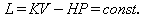(38.1)

So let's try a general approach to macroeconomics. From the Noether-theorems it is known that invariance under time translations always has the importance of an energy. This is now trivial in this simplest form of the quantity equation and thus we get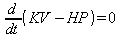and thus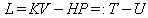With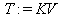And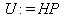(38.2)

That is, the kinetic energy of our system is given by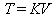and through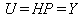the potential energy is given. The four Euler-Lagrange equations are now providing only the mathematically correct though, but useless, trivial solution is identically zero:and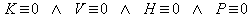(38.3)

The reason is that this is not a conservative force field. This is due to the helical symmetry and thus the fact that a closed round in the force field returns not identical to the first condition. The reason lies in the theorem that follows from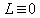this that the total energyremains constant. In our system, but L is not identically zero, and our total energy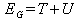(38.4)

increases with time. For nontrivial solutions, one has to divide the quantity equation into suitable sub-variables which accounts for their mutual dependencies differentially. GDP value-free money creation also leads to more so-called anholonom Lagrangians, which are characterized by an additional external source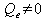: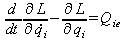(38.5)

In the general case it is to sum over all j products of the real economy, and to sum over the i+k products in the financial industry. This can not possibly backed by GDP contributions alone, but represent a rotational contribution to the total field:And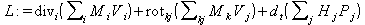(38.6)

to determine from the context of the Euler-Lagrange equations the equations of motion of the full nonlinear system of economics.